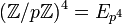Group cohomology of elementary abelian group of prime-square order

Suppose$p$ is a prime number. We are interested in the elementary abelian group of prime-square order$E_{p^2} = (\mathbb{Z}/p\mathbb{Z})^2 = \mathbb{Z}/p\mathbb{Z} \oplus \mathbb{Z}/p\mathbb{Z}$.

Homology groups

Over the integers$H_q(\mathbb{Z}/p\mathbb{Z} \oplus \mathbb{Z}/p\mathbb{Z};\mathbb{Z}) = \left\lbrace \begin{array}{rl} (\mathbb{Z}/p\mathbb{Z})^{(q + 3)/2} & \qquad q = 1,3,5,\dots \\ (\mathbb{Z}/p\mathbb{Z})^{q/2}, & q = 2,4,6,\dots \\ \mathbb{Z}, & \qquad q = 0 \\\end{array}\right.$

The first few homology groups are given below:$q$$0$$1$$2$$3$$4$$5$$H_q$$\mathbb{Z}$ $Z})^2 = E_{p^2$$\mathbb{Z}/p\mathbb{Z}$$(\mathbb{Z}/p\mathbb{Z})^3 = E_{p^3}$$(\mathbb{Z}/p\mathbb{Z})^2 = E_{p^2}$$(\mathbb{Z}/p\mathbb{Z})^4 = E_{p^4}$
rank of$H_q$ as an elementary abelian$p$-group -- 2 1 3 2 4

Over an abelian group

The homology groups with coefficients in an abelian group$M$ are given as follows:$H_q(\mathbb{Z}/p\mathbb{Z} \oplus \mathbb{Z}/p\mathbb{Z};M) = \left\lbrace\begin{array}{rl} (M/pM)^{(q+3)/2} \oplus (\operatorname{Ann}_M(p))^{(q-1)/2}, & \qquad q = 1,3,5,\dots\\ (M/2M)^{q/2} \oplus (\operatorname{Ann}_M(p))^{(q+2)/2}, & \qquad q = 2,4,6,\dots \\ M, & \qquad q = 0 \\\end{array}\right.$

Here,$M/pM$ is the quotient of$M$ by$2M = \{ px \mid x \in M \}$ and$\operatorname{Ann}_M(p) = \{ x \in M \mid px = 0 \}$.

These homology groups can be computed in terms of the homology groups over integers using the universal coefficients theorem for group homology.

Cohomology groups for trivial group action

Over the integers

The cohomology groups with coefficients in the integers are given as below:$H^q(\mathbb{Z}/p\mathbb{Z} \oplus \mathbb{Z}/p\mathbb{Z};\mathbb{Z}) = \left\lbrace \begin{array}{rl} (\mathbb{Z}/p\mathbb{Z})^{(q-1)/2}, & q = 1,3,5,\dots \\ (\mathbb{Z}/p\mathbb{Z})^{(q+2)/2}, & q = 2,4,6,\dots \\ \mathbb{Z}, & q = 0 \\\end{array}\right.$

The first few cohomology groups are given below:$q$$\! 0$$\! 1$$\! 2$$\! 3$$\! 4$$\! 5$$H^q$$\mathbb{Z}$ 0$(\mathbb{Z}/p\mathbb{Z})^2 = E_{p^2}$$\mathbb{Z}/p\mathbb{Z}$$(\mathbb{Z}/p\mathbb{Z})^3 = E_{p^3}$$(\mathbb{Z}/p\mathbb{Z})^2 = E_{p^2}$
rank of$H^q$ as an elementary abelian$p$-group -- 0 2 1 3 2

Over an abelian group

The cohomology groups with coefficients in an abelian group$M$ are given as follows:$H^q(\mathbb{Z}/p\mathbb{Z} \oplus \mathbb{Z}/p\mathbb{Z};M) = \left\lbrace \begin{array}{rl} (\operatorname{Ann}_M(p))^{(q+3)/2} \oplus (M/pM)^{(q-1)/2}, & q = 1,3,5,\dots \\ (\operatorname{Ann}_M(p))^{q/2} \oplus (M/pM)^{(q+2)/2}, & q = 2,4,6,\dots \\ M, & q = 0 \\\end{array}\right.$

Here,$M/pM$ is the quotient of$M$ by$pM = \{ px \mid x \in M \}$ and$\operatorname{Ann}_M(p) = \{ x \in M \mid px = 0 \}$.

These can be deduced from the homology groups with coefficients in the integers using the dual universal coefficients theorem for group cohomology.